Circumference and circle to print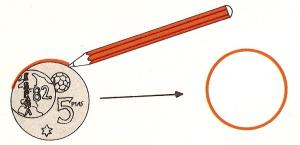Name: ______________________________________   Subject: _______________________  Date: _______

Write the missing word

1. The Circumference
If we put a coin on a paper and we pass a pencil around its edge, we obtain a circumference. With a compass we can also draw it. The compass needle is the center.
The circumference is a closed curve on which all points are at the same distance from its center.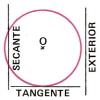2. Circumference parts
The radius is a line segment joining the centre of the circle to any point on the circle.
The diameter is any straight line segment that passes through the center of the circle. It is equivalent to two radiuses. The diameter divides the circumference into two semi-circumferences.
The chord is a line segment whose endpoints lie on the circle.
The arc is a portion of the circumference.
The Secant intersects two points on the curve.
The tangent is the straight line that "just touches" the curve at a single point.
The line that does not touch the circumference is called exterior.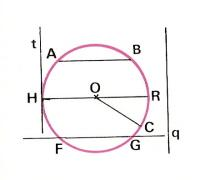Look at the picture and answer these questions:

 The point O is a... The segment OC is a... The segment HR is a... The segment AB is a... The line t is ... The line FG is ...es... The line q is ...es... The Part of the circumference between A and B is... The Part of the circumference between HABR is...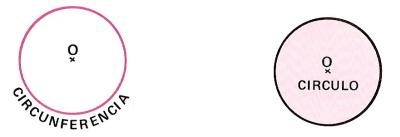3. The circle

The circumference is a line. The ring is an example of circumference.
The circle is a portion of the flat surface bounded by the circumference.
It has two dimensions. The obverse of a coin is an example of circle.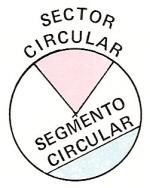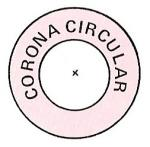4. Circle parts
The diameter divides the circle into two semicircles.
The portion of a disk enclosed by two radii and an arc is called circular sector. If it comprises a quarter of the circle, it is called quadrant.
The annulus is the space between two concentric circles (they have the same center).Look at these pictures and answer these questions:

 Figure 1 is a/an... Figure 2 is a/an... Figure 3 is a/an... Figure 4 is a/an... Figure 5 is a/an... Figure 6 is a/an... Figure 7 is a/an... Figure 8 is a/an...

| Educational applications | Mathematics |
In Spanish  | Interactive

«Arturo Ramo GarcĒa.-Record of intellectual property of Teruel (Spain) No 141, of 29-IX-1999
Plaza Playa de Aro, 3, 1║ DO 44002-TERUEL